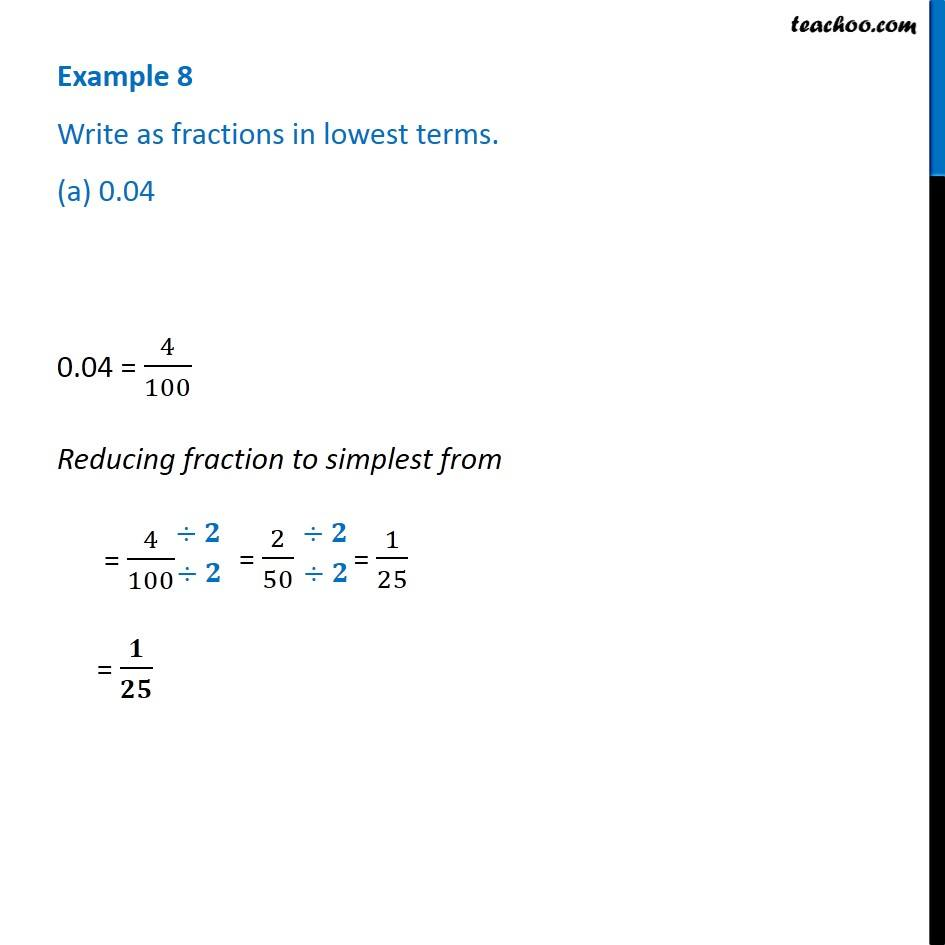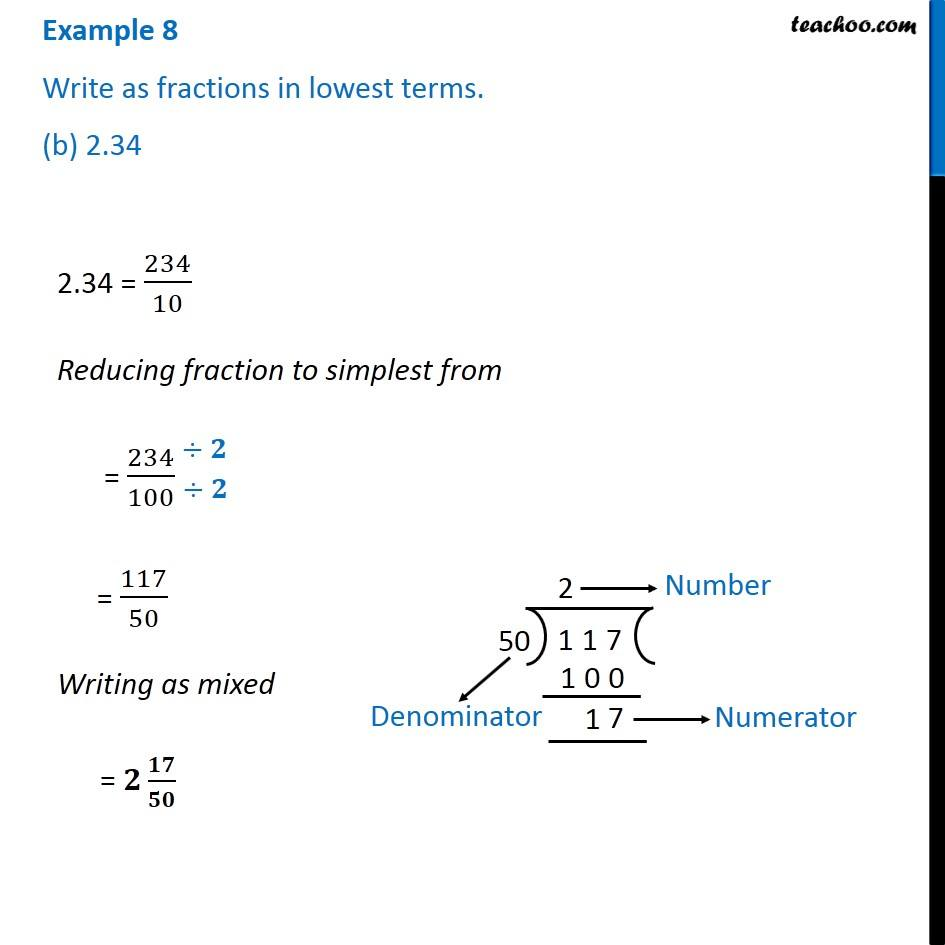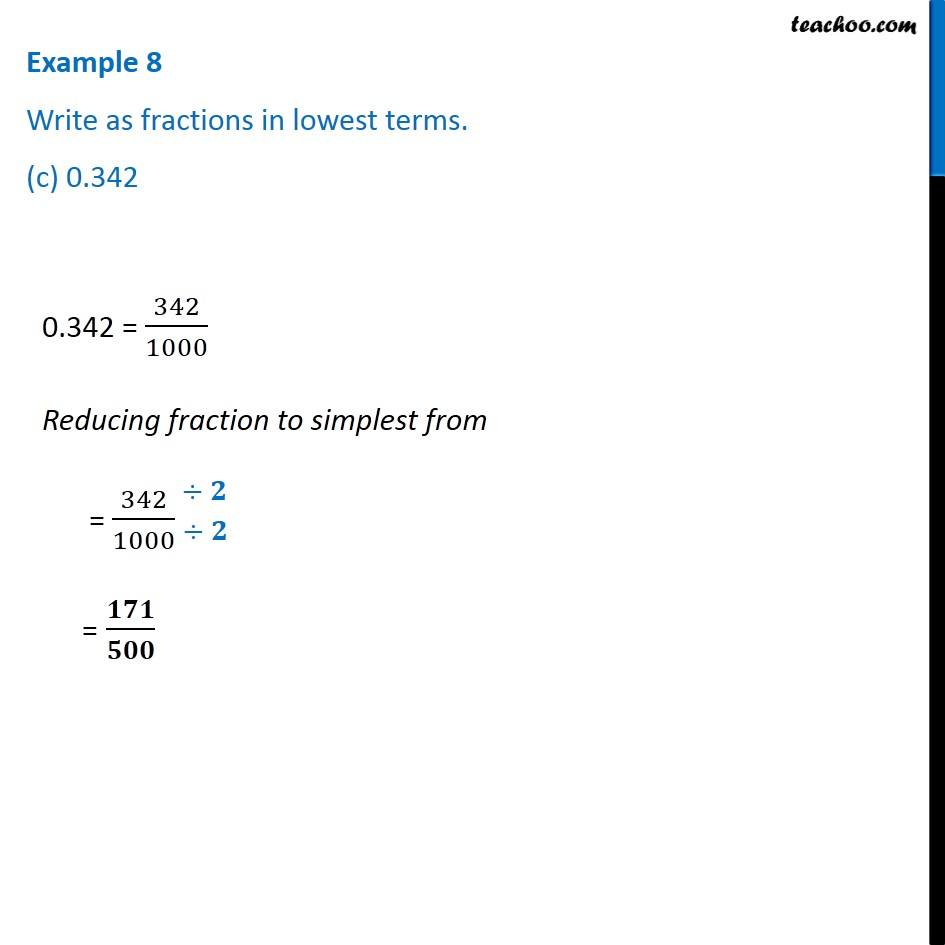Subscribe to our Youtube Channel - https://you.tube/teachoo

1. Chapter 8 Class 6 Decimals
2. Serial order wise
3. Examples

Transcript

Example 8 Write as fractions in lowest terms. (a) 0.04 0.04 = 4/100 Reducing fraction to simplest from = 4/100 = 𝟏/𝟐𝟓 Example 8 Write as fractions in lowest terms. (b) 2.34 2.34 = 234/10 Reducing fraction to simplest from = 234/100 = 117/50 Writing as mixed = 𝟐 𝟏𝟕/𝟓𝟎 Example 8 Write as fractions in lowest terms. (c) 0.342 0.342 = 342/1000 Reducing fraction to simplest from = 342/1000 = 𝟏𝟕𝟏/𝟓𝟎𝟎

Examples

Chapter 8 Class 6 Decimals
Serial order wise